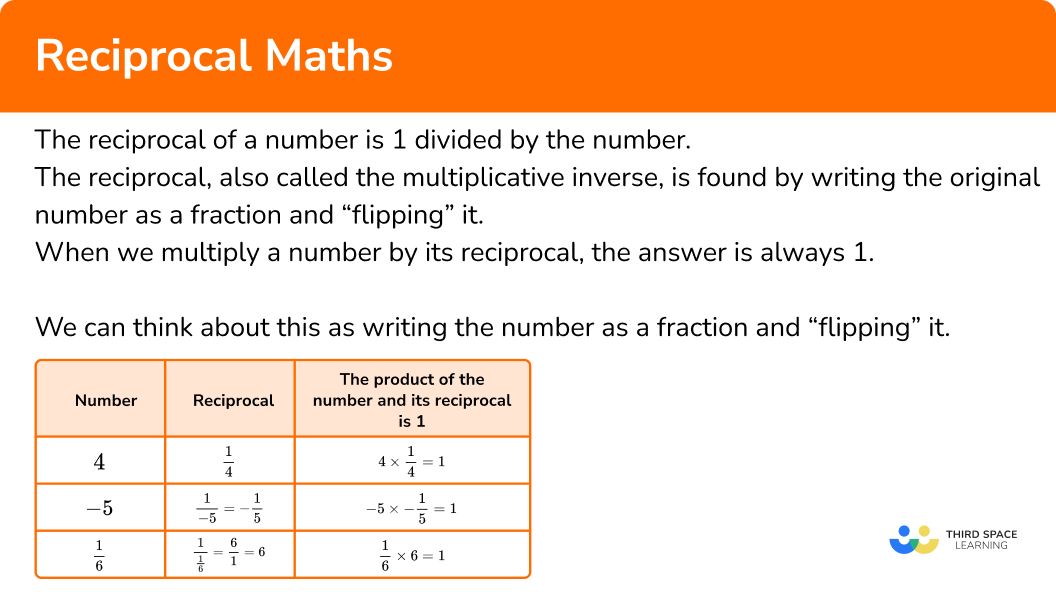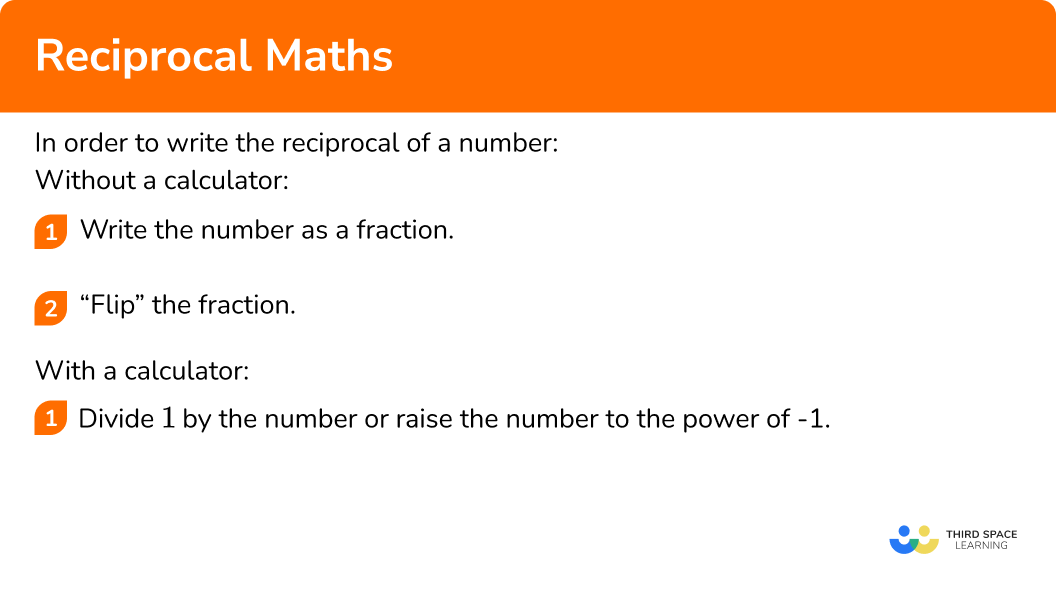# Reciprocal Maths

Here we will learn about reciprocals, including the definition of reciprocal and how to find reciprocals.

There are also reciprocal worksheets based on Edexcel, AQA and OCR exam questions, along with further guidance on where to go next if you’re still stuck.

## What is a reciprocal?

The reciprocal of a number is 1 divided by the number. It can also be found by raising the number to the power of -1 .

The reciprocal, also called the multiplicative inverse, is found by writing the original number as a fraction and “flipping” it.

When we multiply a number by its reciprocal, the answer is always 1.

For example,

Every number has a reciprocal except 0 \ (1 \div 0 is undefined).

Given that the method of finding a reciprocal is to flip the number when it is written as a fraction, you might notice that the reciprocal of a reciprocal is the original number.

For example, the reciprocal of 5 is \frac{1}{5} and the reciprocal of \frac{1}{5} is \frac{5}{1}=5.

### What is a reciprocal?### Reciprocal graphs

Reciprocal functions produce distinctive looking graphs. Here is the graph of y=\frac{1}{x}, the basic reciprocal function.

Notice that the graph is undefined for x=0 since we cannot calculate 1 \div 0.

Step-by-step guide: Reciprocal graphs

## How to write the reciprocal of a number

In order to write the reciprocal of a number:

Without a calculator:

1. Write the number as a fraction.
2. “Flip” the fraction.

Alternatively, if you have a calculator:

1. Divide \bf{1} by the number or raise the number to the power of \bf{-1}.

### Explain how to write the reciprocal of a number## Reciprocal maths examples

### Example 1: reciprocal of a whole number

Write down the reciprocal of 7.

1. Write the number as a fraction.

First we write the number 7 as a fraction. 7 is the numerator and 1 is the denominator.

7=\frac{7}{1}.

2“Flip” the fraction.

Then we can “flip” the fraction by inverting it, or turning it upside down.

So the reciprocal of the number 7 is

\frac{1}{7}.

### Example 2: reciprocal of a fraction

Write down the reciprocal of \frac{2}{3}.

Write the number as a fraction.

“Flip” the fraction.

### Example 3: reciprocal of an improper fraction

Write down the reciprocal of \frac{9}{5}.

Write the number as a fraction.

“Flip” the fraction.

### Example 4: reciprocal of a mixed number

Write down the reciprocal of 3\frac{1}{2}.

Write the number as a fraction.

“Flip” the fraction.

### Example 5: reciprocal of a decimal number less than 1

Write down the reciprocal of 0.3.

Write the number as a fraction.

“Flip” the fraction.

### Example 6: reciprocal of a decimal number greater than 1

Write down the reciprocal of 4.8.

Write the number as a fraction.

“Flip” the fraction.

### Example 7: reciprocal of a negative number

Write down the reciprocal of -3.

Write the number as a fraction.

“Flip” the fraction.

### Common misconceptions

• Reciprocal of zero

Zero does not have a reciprocal. This is because 1 \div 0 is undefined (it does not exist).

• Fractions and decimals

Whilst some fractions can easily be written as decimals, many fractions cannot be written as exact decimals. When this is the case, leave your answer as a fraction as it is more accurate.

\frac{1}{7} cannot be written as an exact decimal so leave your answer as a fraction.

### Practice reciprocal maths questions

1. Find the reciprocal of 9.

1.9-\frac{1}{9}\frac{1}{9}0.9To find the reciprocal of 9 we first write it as a fraction with 1 as the denominator. Then we turn the fraction upside down.

9=\frac{9}{1}

So the reciprocal is \frac{1}{9}.

2. Find the reciprocal of \frac{6}{11}.

6.11\frac{11}{6}-\frac{11}{6}11.6To find the reciprocal we turn the fraction upside down.

So the reciprocal is \frac{11}{6}.

3. Find the reciprocal of \frac{8}{5}.

0.62562.5\frac{8}{13}\frac{5}{13}To find the reciprocal we turn the fraction upside down.

So the reciprocal is \frac{5}{8}.

As a decimal this is 0.625.

4. Find the reciprocal of 4\frac{1}{5}.

4.15\frac{41}{5}\frac{5}{21}41.5We first need to write this mixed number as an improper fraction. Then we can turn the fraction upside down.

4\frac{1}{5}=\frac{21}{5}

So the reciprocal is \frac{5}{21}.

5. Find the reciprocal of 0.8.

1.21.251.38To find the reciprocal of 0.8 we first write it as a fraction. Then we turn the fraction upside down.

0.8=\frac{8}{10}

So the reciprocal is \frac{10}{8}.

This can be written as 1.25.

6. Find the reciprocal of 5.6.

6.5\frac{28}{5}-5.6\frac{5}{28}To find the reciprocal of 5.6 we first write it as an improper fraction. Then we turn the fraction upside down.

5.6=5\frac{6}{10}=\frac{56}{10}

So the reciprocal is \frac{10}{56}.

This can be simplified to \frac{5}{28}.

### Reciprocal maths GCSE questions

1. Write down the reciprocal of \frac{1}{8} .

(1 mark)

8

(1)

2. Find the reciprocal of \frac{3}{11} . Write your answer as a mixed number.

(2 marks)

\frac{11}{3}

(1)

3\frac{2}{3}

(1)

3. Find the reciprocal of 3.1.

(2 marks)

3.1=\frac{31}{10}

(1)

\frac{10}{31}

(1)

## Learning checklist

You have now learned how to:

• Find the reciprocal of an integer
• Find the reciprocal of a fraction or a decimal number

## Still stuck?

Prepare your KS4 students for maths GCSEs success with Third Space Learning. Weekly online one to one GCSE maths revision lessons delivered by expert maths tutors.

Find out more about our GCSE maths tuition programme.